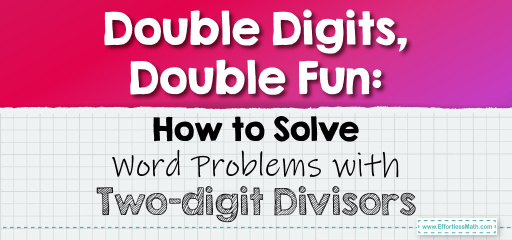# Double Digits, Double Fun: How to Solve Word Problems with Two-digit Divisors

Real-life scenarios often present us with situations where we need to divide two-digit or three-digit numbers by two-digit numbers. These word problems can range from sharing resources to calculating averages. Let's explore some word problems that involve this type of division.## Dividing Two-digit and Three-digit Numbers by Two-digit Numbers in Word Problems

### Example 1:

A school ordered $$240$$ pencils and wants to distribute them equally among $$20$$ classrooms. How many pencils will each classroom receive?

Solution Process:

Divide $$240$$ by $$20$$.

Each classroom will receive $$12$$ pencils.

The Absolute Best Book for 5th Grade Students

### Example 2:

There are $$154$$ students in a school who want to play soccer. If each team requires $$22$$ players, how many full teams can the school form?

Solution Process:

Divide $$154$$ by $$22$$.

The school can form $$7$$ full teams with $$0$$ students left over.

Dividing two-digit and three-digit numbers by two-digit numbers in word problems requires careful reading and understanding of the problem’s context. By identifying the numbers involved and performing the division, you can find the solution and apply this knowledge to various real-world scenarios. Whether it’s distributing resources, forming groups, or calculating averages, division plays a pivotal role. Practice regularly with different word problems to become adept at using division in practical situations!

### Practice Questions:

1. A bakery baked $$360$$ muffins and wants to pack them in boxes of $$24$$. How many full boxes will they have?

2. A library has $$528$$ books and wants to distribute them equally among $$33$$ tables. How many books will each table get?

3. There are $$275$$ students going on a field trip. If each bus can carry $$25$$ students, how many buses are needed?

4. A factory produces $$462$$ toys and wants to pack them in boxes of $$21$$. How many boxes will they prepare?

5. A marathon event has $$540$$ water bottles to be distributed equally among $$45$$ hydration stations. How many bottles will each station get?

A Perfect Book for Grade 5 Math Word Problems!

1. $$15$$ full boxes

2. $$16$$ books per table with a remainder of $$0$$

3. $$11$$ buses

4. $$22$$ boxes

5. $$12$$ bottles per station

The Best Math Books for Elementary Students

### What people say about "Double Digits, Double Fun: How to Solve Word Problems with Two-digit Divisors - Effortless Math: We Help Students Learn to LOVE Mathematics"?

No one replied yet.

X
51% OFF

Limited time only!

Save Over 51%

SAVE $15 It was$29.99 now it is \$14.99